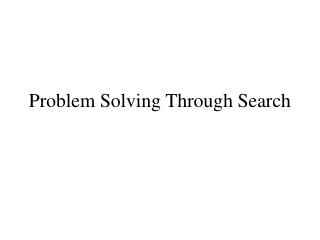DownloadDownload PresentationProblem Solving Through Search

# Problem Solving Through Search

Télécharger la présentation## Problem Solving Through Search

- - - - - - - - - - - - - - - - - - - - - - - - - - - E N D - - - - - - - - - - - - - - - - - - - - - - - - - - -
##### Presentation Transcript

1. Problem Solving Through Search

2. Problems and Search • Problem formulation: an essential step in building an intelligent agent • Search: a fundamental and very common solution technique • Chapter 3: “Goal”-oriented problems, and basic search strategies • Chapter 4: “Informed” search strategies

3. Problem Formulation • Basic elements of problem formulation: • The states • The initial state • The Goalstate(s) (note: may need a goal test) • Actions available at each states • Solution: a path from initial to a goal • In general, an abstraction of a more complicated problem

4. Example Problems • Traveling from one city to another • The states: the cities • The actions: available direct paths from one city to another • Initial state: start city • Goal state: destination city • Solution: Sequence of states (actions) connecting initial to the goal

5. Example Problems (cont.) • That was simple! (still non-trivial abstraction) • The 8 puzzle (states? actions?) • The 8-queens • The vacuum world • “Real-world:” Robot navigation, VLSI layout, touring and traveling salesperson problems

6. Possible Extensions/Complications • Consider path cost too • Uncertainty about the current (e.g. initial) state (multiple-state problems, e.g. robotics) • Uncertainty or even minimal knowledge about the effects of actions (e.g. robotics) • Contingencies, exogenous events • Continuous problems (states and actions)

7. Problem Solving Performance • Cost of coming up with a solution (computational costs, e.g. search cost) • Cost of executing the solution

8. Solution Technique: SEARCH • Search through the state space • Maintain and extend a set of partial solution sequences • Offline, simulated exploration of state space • “expand” a state to generate next states • Search strategy: the order/criterion of which state to expand next

9. Search Trees • Visualization and implementation aid: search tree • Tree nodes correspond to states • Note: Search tree different from state space (e.g. search trees can be arbitrarily big, while the state space could be finite) • Queue: a valuable data structure for implementing various search strategies

10. Some Search Strategies • Breadth first (BF) • Uniform Cost (generalization of BF) • Depth first • Iterative deepening search • Depth limited • Bidirectional

11. Evaluating Search Strategies • Evaluated based on: • Completeness (always finds a solution?) • Time complexity (how long to find a solution?) • Space complexity (how much memory?) • Optimality (always finds the highest quality solution?) • Parameters • b -- maximum or average branching factor • d – depth of the least cost solution • m – maximum depth of state space

12. Breadth First • States are expanded “horizontally” • How would this work with a queue implementation? • Properties: Complete? Time? Space? Optimal? • Space versus time costs in practice

13. Uniform Cost Search • Uniform-Cost Search: expand states by their path distance (cost) to root node • Connection to BF search? • Properties?

14. Depth First • Expand “vertically” or expand the deepest • Properties? • Less memory (linear with depth) • Can find a solution faster than BF (e.g. when many goal states appear) • Easily implemented with recursion

15. Depth Limited and Iterative Deepening • Depth-limited search: depth first search, but with a bound on depth explored • Iterative deepening: depth-limited but successively increase the bound until a goal is found • Properties?

16. Bidirectional Search • Do search from the start state and (backwards) from a goal state until they meet • Some requirements: • Need to have a goal state identifiable (what if there are too many?) • Predecessor states can be generated (easily) • Why could it be better?

17. Summary • Problem formulation requires abstraction, and to be amenable to search techniques, requires identification of states and actions • Search is a very general solution methodology • Variety of uninformed search algorithms • Several useful criteria for comparing search technqiues